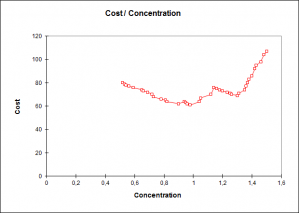# ROC curves

ROC curves measure the efficiency of a binary classifier using sensitivity and specificity. Available in Excel using the XLSTAT add-on statistical software.The ROC curve generated by XLSTAT allows to represent the evolution of the proportion of true positive cases (also called sensitivity) as a function of the proportion of false positives cases (corresponding to 1 minus specificity), and to evaluate a binary classifier such as a test to diagnose a disease, or to control the presence of defects on a manufactured product.

## What is a ROC curve?

The ROC curve corresponds to the graphical representation of the couple (1 – specificity, sensitivity) for the various possible threshold values.

Here are some important definitions:

• Sensitivity (equivalent to the True Positive Rate): Proportion of positive cases that are well detected by the test. In other words, the sensitivity measures how the test is effective when used on positive individuals. The test is perfect for positive individuals when sensitivity is 1, equivalent to a random draw when sensitivity is 0.5. If it is below 0.5, the test is counter-performing and it would be useful to reverse the rule so that sensitivity is higher than 0.5 (provided that this does not affect the specificity). The mathematical definition is given by: Sensitivity = TP/(TP + FN).
• Specificity (also called True Negative Rate): proportion of negative cases that are well detected by the test. In other words, specificity measures how the test is effective when used on negative individuals. The test is perfect for negative individuals when the specificity is 1, equivalent to a random draw when the specificity is 0.5. If it is below 0.5, the test is counter-performing and it would be useful to reverse the rule so that specificity is higher than 0.5 (provided that this does not affect the sensitivity). The mathematical definition is given by: Specificity = TN/(TN + FP).

## Area Under the Curve

The area under the curve (AUC) is a synthetic index calculated for ROC curves. The AUC is the probability that a positive event is classified as positive by the test given all possible values of the test. For an ideal model we have AUC = 1 (above in blue), where for a random pattern we have AUC = 0.5 (above in red). One usually considers that the model is good when the value of the AUC is higher than 0.7. A well discriminating model should have an AUC between 0.87 and 0.9. A model with an AUC above 0.9 is excellent.

Sen (1960), Bamber (1975) and Hanley and McNeil (1982) have proposed different methods to calculate the variance of the AUC. All are available in XLSTAT. XLSTAT offers as well a comparison test of the AUC to 0.5, the value 0.5 corresponding to a random classifier. This test is based on the difference between the AUC and 0.5 divided by the variance calculated according to one of the three proposed methods. The statistic obtained is supposed to follow a standard normal distribution, which allows the calculation of the p-value.

The AUC can also be used to compare different tests between them. If the different tests have been applied to different groups of individuals, samples are independent. In this case, XLSTAT uses a Student test to compare the AUCs (which requires assuming the normality of the AUC, which is acceptable if the samples are not too small). If different tests were applied to the same individuals, the samples are paired. In this case, XLSTAT calculates the covariance matrix of the AUCs as described by Delong and Delong (1988) on the basis of Sen’s work (1960), to then calculate the variance of the difference between two AUCs, and to calculate the p-value assuming the normality.

## XLSTAT results for the ROC analysis

In addition to the ROC and AUC curve, other results are computed.

### ROC analysis

The ROC analysis table displays for each possible threshold value of the test variable, the various indices presented in the description section. On the line below the table you'll find a reminder of the rule set out in the dialog box to identify positive cases compared to the threshold value. Below the table you will find a stacked bars chart showing the evolution of the TP, TN, FP, FN depending on the value of the threshold value. If the corresponding option was activated, the decision plot is then displayed (for example, changes in the cost depending on the threshold value).

### Comparison of the AUC to 0.5

These results allow to compare the test to a random classifier. The confidence interval corresponds to the difference. Various statistics are then displayed including the p-value, followed by the interpretation of the comparison test.

### Comparison of the AUCs

If you selected several test variables, once the above results are displayed for each variable, you will find the covariance matrix of the AUC, followed by the table of differences for each pair of AUCs with as comments the confidence interval, and then the table of the p-values. Values in bold correspond to significant differences. Last, a graph that compares the ROC curves displayed.### analyze your data with xlstat

14-day free trial

Included in

Related features